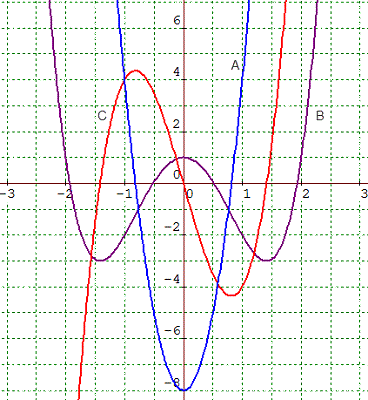Joy C.

The graph below shows the graph of f (x), its derivative f '(x), and its second derivative f "(x). Which of the following is the correct statement?(4 points)

 A is f ', B is f, C is f ". I chose this answer: A is f ", B is f, C is f '. A is f ', B is f ". C is f. A is f, B is f ', C is f ".Mark M.

Report

10/23/20